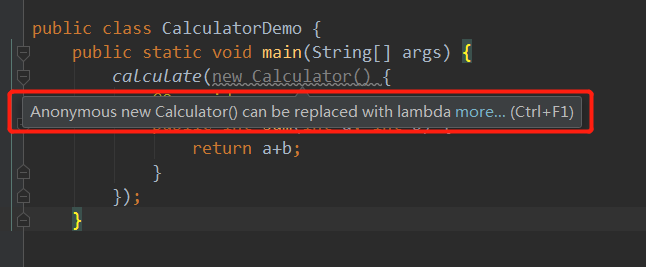# 学习lambda函数, 这一篇就够了

1.我们知道, 在java8之前, 接口由两个部分组成, 抽象方法和常量(成员变量默认修饰符public static final, 成员方法默认修饰符public absolute)

1.抽象方法   2.常量  3.默认方法(Java8)  4.静态方法(java8)  5.私有方法(java9)

/**
* 由于本人使用的是java8, 故私有方法不能演示, 有兴趣的同学可以试一试
*
* @author wenfucheng
*/
public interface Calculator {

default void defaultMethod(){
System.out.println("接口中的默认方法");
}

static void staticMethod(int a, int b){
System.out.println("接口中的静态方法");
}

int sum (int a, int b);
}

public class CalculatorDemo {
public static void main(String[] args) {
calculate(new Calculator() {
@Override
public int sum(int a, int b) {
return a+b;
}
});
}

public static void calculate(Calculator calculator){
System.out.println(calculator.sum(10,20));
}
}public static void main(String[] args) {
// 原始版
calculate((int x,int y) ->{return x+y;});
// 精简版
calculate((x,y) -> x+y);
}

public static void main(String[] args) {
}

@FunctionalInterface

public interface 函数式接口名称{...

}

@FunctionalInterface
public interface Calculator {

default void defaultMethod(){
System.out.println("接口中的默认方法");
}

static void staticMethod(int a, int b){
System.out.println("接口中的静态方法");
}

int sum (int a, int b);
}

/**
* 需求: 将list里面的字符串切割, 分离数字和字符串, 然后将字符串转成数字,
*       然后过滤出数字大于20的, 最后再转成一个集合
*/

public static void main(String[] args) {
List<String> list = Arrays.asList("11,北京","22,上海","33,广州","44,深圳");

/**
* 通过stream流改造后的list为[22,33,44]
*/
List<Integer> collect = list.stream().map(s -> s.split(",")).
map(Integer::parseInt).filter(s -> s > 20).collect(Collectors.toList());

/**
* 通过parallelStream(并发流), 效率会更高
*/
List<Integer> collect1 = list.parallelStream().map(s -> s.split(",")).
map(Integer::parseInt).filter(s -> s > 20).collect(Collectors.toList());
}

filter(过滤) 传递一个返回boolean类型的函数

collect 将流转成集合